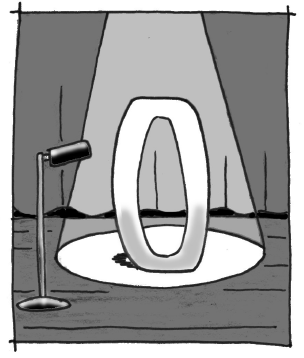### Home > INT2 > Chapter 5 > Lesson 5.1.2 > Problem5-21

5-21.

1. Copy each problem and calculate each sum:

$0+3=$

$-7+0=$

$0+6=$

$0+(-2)=$

1. What is special about adding zero? Write a sentence that begins, “When you add zero to a number, …”

1. Julia is thinking of two numbers, $a$ and $b$. When she adds them together, she gets a sum of $b$. Does that tell you anything about either of Julia’s numbers?

What did you notice about each of the answers in part (a)?1. Copy each problem and calculate each product:

$3\cdot0=$

$(-7)\cdot0=$

$0\cdot6=$

$0\cdot(-2)=$

1. What is special about multiplying by zero? Write a sentence that begins, “When you multiply a number by zero, …”

When you multiply a number by $0$, … the result is always $0$.

2. Julia is thinking of two numbers, $a$ and $b$. When she multiplies them together, she gets a product of $0$. Does that tell you anything about either of Julia’s numbers?